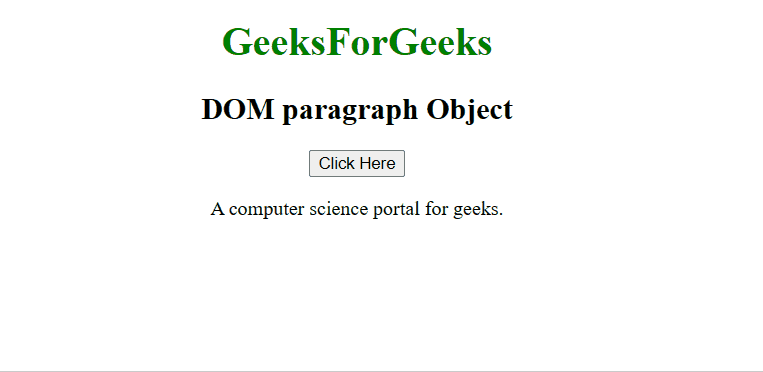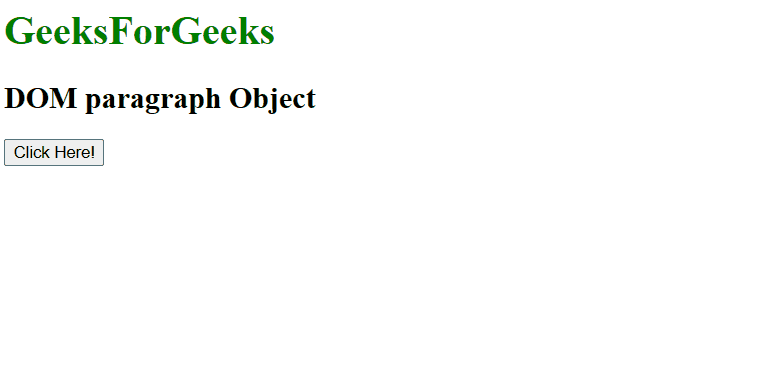# HTML DOM Paragraph Object

The DOM paragraph object is used to represent the HTML <p> element. The p element is accessed by getElementById().

Syntax:

`document.getElementById("id"); `

Where ‘id’ is the ID assigned to the p tag.

The below programs illustrate the p object:

Property Values

• align: It is used to set or return the alignment of the paragraph element.

Example 1: In the below program the paragraph element is assigned the id = “s”. With the click of the button, the paragraph element is accessed and the color of the paragraph text is changed.

## HTML

 `` `<``html``>`   `<``body``>` `    ``<``h1` `style``=``"color:green;"``>` `        ``GeeksForGeeks` `    ``` `    ``<``h2``>DOM paragraph Object` `    ``<``button` `onclick``=``"Geeks()"``>` `        ``Click Here` `    ``` `    ``<``p` `id``=``"s"``>` `        ``A computer science` `        ``portal for geeks.` `    ```   `    ``<``script``>` `        ``function Geeks() {` `            ``let txt = document.getElementById("s");` `            ``txt.style.color = "green";` `        ``}` `    ``` ``   ``

Output:Example 2: A paragraph Object can be created by using the document.createElement method. In the below program, we create a paragraph element and append a text node to it.

## HTML

 `` `<``html``>`   `<``body``>` `    ``<``h1` `style``=``"color:green;"``>` `        ``GeeksForGeeks` `    ``` `    ``<``h2``>DOM paragraph Object` `    ``<``button` `onclick``=``"Geeks()"``>` `        ``Click Here!` `    ``` `    ``<``br``><``br``>`   `    ``<``div` `id``=``"p"``>` `    `  `    ``<``script``>` `        ``function Geeks() {` `            ``let txt = document.createElement("P");` `            ``let t = document.createTextNode(` `                ``"A computer science portal for geeks.");` `            ``txt.appendChild(t);` `            ``document.getElementById("p").appendChild(txt);` `        ``}` `    ``` ``   ``

Output:Supported Browsers: